# Sparse Spikes Deconvolution with MUSIC Algorithm¶

Important: Please read the installation page for details about how to install the toolboxes. $\newcommand{\dotp}{\langle #1, #2 \rangle}$ $\newcommand{\enscond}{\lbrace #1, #2 \rbrace}$ $\newcommand{\pd}{ \frac{ \partial #1}{\partial #2} }$ $\newcommand{\umin}{\underset{#1}{\min}\;}$ $\newcommand{\umax}{\underset{#1}{\max}\;}$ $\newcommand{\umin}{\underset{#1}{\min}\;}$ $\newcommand{\uargmin}{\underset{#1}{argmin}\;}$ $\newcommand{\norm}{\|#1\|}$ $\newcommand{\abs}{\left|#1\right|}$ $\newcommand{\choice}{ \left\{ \begin{array}{l} #1 \end{array} \right. }$ $\newcommand{\pa}{\left(#1\right)}$ $\newcommand{\diag}{{diag}\left( #1 \right)}$ $\newcommand{\qandq}{\quad\text{and}\quad}$ $\newcommand{\qwhereq}{\quad\text{where}\quad}$ $\newcommand{\qifq}{ \quad \text{if} \quad }$ $\newcommand{\qarrq}{ \quad \Longrightarrow \quad }$ $\newcommand{\ZZ}{\mathbb{Z}}$ $\newcommand{\CC}{\mathbb{C}}$ $\newcommand{\RR}{\mathbb{R}}$ $\newcommand{\EE}{\mathbb{E}}$ $\newcommand{\Zz}{\mathcal{Z}}$ $\newcommand{\Ww}{\mathcal{W}}$ $\newcommand{\Vv}{\mathcal{V}}$ $\newcommand{\Nn}{\mathcal{N}}$ $\newcommand{\NN}{\mathcal{N}}$ $\newcommand{\Hh}{\mathcal{H}}$ $\newcommand{\Bb}{\mathcal{B}}$ $\newcommand{\Ee}{\mathcal{E}}$ $\newcommand{\Cc}{\mathcal{C}}$ $\newcommand{\Gg}{\mathcal{G}}$ $\newcommand{\Ss}{\mathcal{S}}$ $\newcommand{\Pp}{\mathcal{P}}$ $\newcommand{\Ff}{\mathcal{F}}$ $\newcommand{\Xx}{\mathcal{X}}$ $\newcommand{\Mm}{\mathcal{M}}$ $\newcommand{\Ii}{\mathcal{I}}$ $\newcommand{\Dd}{\mathcal{D}}$ $\newcommand{\Ll}{\mathcal{L}}$ $\newcommand{\Tt}{\mathcal{T}}$ $\newcommand{\si}{\sigma}$ $\newcommand{\al}{\alpha}$ $\newcommand{\la}{\lambda}$ $\newcommand{\ga}{\gamma}$ $\newcommand{\Ga}{\Gamma}$ $\newcommand{\La}{\Lambda}$ $\newcommand{\si}{\sigma}$ $\newcommand{\Si}{\Sigma}$ $\newcommand{\be}{\beta}$ $\newcommand{\de}{\delta}$ $\newcommand{\De}{\Delta}$ $\newcommand{\phi}{\varphi}$ $\newcommand{\th}{\theta}$ $\newcommand{\om}{\omega}$ $\newcommand{\Om}{\Omega}$

This numerical tour explores the use of the MUSIC algorithm to perform sparse deconvolution.

The MUSIC algorithm was introduced by Schmidt. It is closely related to Prony's method Prony, and is very popular in signal processing KrimViberg, where it is mostly used for line spectral estimation (i.e. find locations of Diracs in the Fourier spectrum), and we re-formulate here as a problem of finding Diracs' over the temporal domain.

We follow here the exposition of LiaoFannjiang who propose a theoretical analysis of the performances of this method. Several related algorithms exists, see for instance RoyKailathHuaSarkar,DemNeedNg.

In :
addpath('toolbox_signal')

In :
ms = 20; % markersize


## MUSIC Algorithm¶

We consider here the problem of recovering a Radon measure $m_0 \in \Mm(\mathbb{T})$ defined on the torus $\mathbb{T}=\RR/\ZZ$ from of possibly noisy low-pass measurements $$y = y_0 + w \qwhereq y_0 = \Phi m_0$$ where $w$ is the noise term, and so that $\Phi m$ computes the first $M+1$ low frequencies of the Fourier transform of $m$, i.e. $$\forall \ell \in \{0,\ldots,M\}, \quad (\Phi m)_\ell = \int_{\mathbb{T}} e^{-2\imath\pi x \ell} d m(x).$$ We only consider positive frequency because we assume $m$ is a real measure.

In the following, we consider a discrete measure of the form $m_0=m_{a_0,x_0}$ where we used the following notation $$m_{a,x} = \sum_{i=1}^N a_i \de_{x_i}$$ where $a \in \RR^N$ and $x \in \mathbb{T}^N$.

We thus have $\Phi m_{a,x} = \Phi_x^M a$ where $$\Phi_x^{M} = ( e^{-2\imath\pi x_j \ell} )_{0 \leq\ell \leq M, 1 \leq j \leq N} \in \RR^{(M+1) \times N}.$$

Note that $\Phi_x^{M}$ is a (rectangular) Vandermonde matrix, since $$\Phi_x^M = (V_{\ga(x)}^M)^* \qwhereq \ga(x) = ( e^{2\imath\pi x_j} )_{j=1}^N \in \CC^N.$$

For $y \in \RR^M$ and for $0<L<M$ we denote the Hankel matrix $$H_y = \begin{pmatrix} y_0 & y_1 & \ldots & y_{M-L} \\ y_1 & y_2 & \ldots & y_{M-L+1} \\ \vdots & \vdots & \ddots & \vdots \\ y_L & y_{L+1} & \ldots & y_M \end{pmatrix} \in \RR^{(L+1) \times (M-L+1) }.$$

Since $y=\Phi_x^M a+w$, one can check that one has the following factorization $$H_y = \Phi_x^{L} \diag(a) ( \Phi_x^{M-L} )^* + H_{w}.$$

We suppose that $\min(L,M-L+1) \geq N$. The fundamental property, which is based on the above factorization, is that $$s \in \{x_{0,1},\ldots,x_{0,N}\} \quad\Longleftrightarrow\quad \phi_L(s) \in \text{Im}(H_{y_0}) \qwhereq \phi_L(s) = (e^{-2\imath\pi \ell s })_{0 \leq s \leq L} \in \CC^{L+1}.$$

From observations $y$, we define the following detection function $$\forall s \in \mathbb{T}, \quad d_y(s) = \norm{ U^{\bot,*} \phi^L(s) }^2,$$ where we considered the following SVD factorization $$H_y = [U, U^\bot] \diag_j(\si_j) [V, V^\bot]^*$$ $$\qwhereq \choice{ U \in \CC^{(L+1) \times N}, U^\bot \in \CC^{(L+1) \times (L+1-N)} \\ V \in \CC^{(M-L+1) \times N}, V^\bot \in \CC^{(M-L+1) \times (M-L+1-N)}. }$$

The detection property can hence be conveniently re-written using this detection function as $$\{x_{0,1},\ldots,x_{0,N}\} = \enscond{ s }{ d_{y_0}(s)=0 }.$$

## Sparse Spikes Deconvolution¶

We compute here some low frequency measurements.

Number $M$ of recorded frequency.

In :
M = 18;


Imaging grid, for display purpose.

In :
Q = 1024*4;
z = (0:Q)'/Q;


Input positions $x_0 \in \mathbb{T}^N$.

In :
x0 = [.1 .4 .5 .7 .9]';


Number $N$ of spikes.

In :
N = 5;


Input amplitudes $a_0 \in \RR^N$.

In :
a0 = [.7 -.8 .9 1 -.9]';


Observation operator at position $x$, i.e. $\Phi_x$.

In :
Phi = @(x)exp(-2i*pi*(0:M)'*x(:)');


Observations $y=y_0+w$.

In :
sigma = .15;
w = (randn(M+1,1)+1i*randn(M+1,1)); w = w/norm(w);
y0 = Phi(x0)*a0;
y = y0 + sigma*norm(y0)*w;


Compute the observed low frequency signal $$f = \frac{1}{2M+1} \sum_{\ell=-P}^P y_\ell e^{2\imath\pi \ell \cdot}$$ where we completed the observation using $y_{-\ell} = \bar y_{\ell}$ since the input measure is real.

In :
PhiT = @(x)exp(2i*pi*x(:)*(-M:M)) / (2*M+1);
f = real( PhiT(z) * [conj(y(end:-1:2)); y]);


Display the observed signal $f$, together with the spikes locations.

In :
clf; hold on;
plot(z, f);
stem(x0,a0, 'r');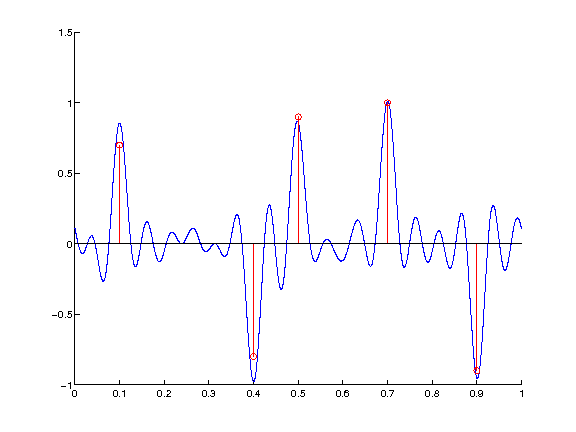## Noiseless Recovery ¶

We first check the exact recovery when using $y_0$.

We set here $L=M/2$, which is a standard choice.

In :
L = M/2;


Build Hankel matrix, one must have min(size(H))>N.

In :
MusicHankel = @(y)hankel(y(1:L),y(L:M));


Compute SVD of $H_{y_0}$.

In :
[U,S,V] = svd(MusicHankel(y0),0);
S = diag(S);


Display the decay of the eigenvalues of $H_{y_0}$. Since there is no noise, only the $N$ largest one are non-zero. This is a convenient way to detect $N$ if it is unknown.

In :
clf;
plot(S, '.-', 'MarkerSize', ms);
axis tight;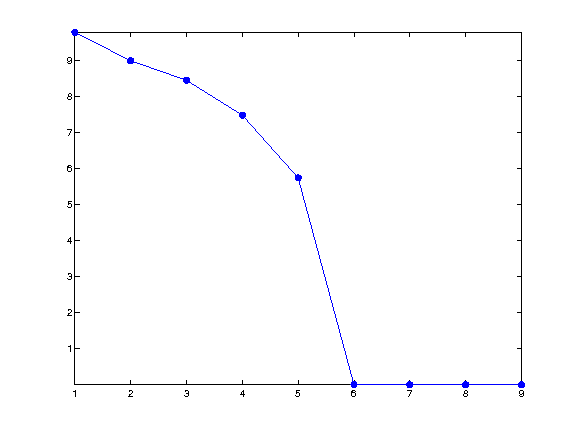Imaging function $d_{y_0}$, evaluated on a thin grid $z \in \mathbb{T}^Q$.

In :
Ubot = U(:,N+1:end);
d = Ubot'*exp(-2i*pi*(0:L-1)'*z(:)');
d = sum(abs(d).^2) / L;


Display $d$.

In :
clf; hold on;
plot(z, d, 'b');
stem(x0, 1+x0*0, 'r.');
axis([0 1 0 1]); box on;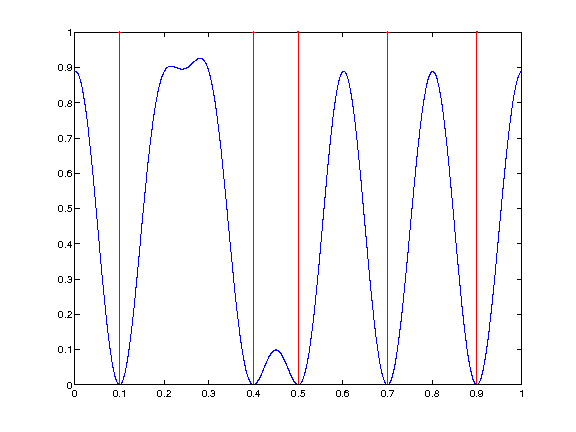## Computing the Diracs' Locations by Root Finding¶

Instead of evaluating the function $d_{y_0}$ on a thin grid, it is possible to compute directly its zeros using root finding.

Indeed, one has $$d_y(s) = \norm{ U^{\bot,*} \phi^L(s) }^2 = \sum_j \sum_{\ell,\ell'} \bar U^{\bot}_{\ell,j} U^{\bot}_{\ell',j} z^{\ell-\ell'}$$ where we denoted $z=e^{2\imath\pi s}$.

So the zeros of $d_y$ are equal to the zeros of the polynomial $P_y$ that are on the unit circle, where we introduced $$P_y(z) = \sum_\ell C_\ell z^\ell \qwhereq \choice{ C_\ell = \sum_{j} B_{j,\ell}, \\ B_{j,\cdot} = U_{\cdot,j} \star \bar U_{-\cdot,j}, \\ }$$ where $\star$ is the product of convolution.

This means that $$\enscond{s}{d_y(s)=0} = \frac{1}{2\pi} \text{Angle}\pa{ \enscond{z}{P_y(z)=0 \qandq \abs{z}=1} }$$ where Angle returns the angle of a complex number.

Compute the coefficients $C$ of the polynomial $P_{y_0}$.

In :
B = [];
for j=1:size(Ubot,2)
u = Ubot(:,j);
v = flipud(conj(u));
B(:,j) = conv(u,v);
end
C = sum(B,2);


Find its roots.

In :
R = roots(C(end:-1:1));


Locate those that are along the circle.

In :
R1 = R(abs( abs(R)-1 )<1e-4);


Display roots.

In :
clf; hold on;
plot(exp(2i*pi*z), 'k');
plot(R, 'b.', 'MarkerSize', ms);
plot(R1, 'r.', 'MarkerSize', ms);
axis equal; box on;
axis([-1 1 -1 1]*2);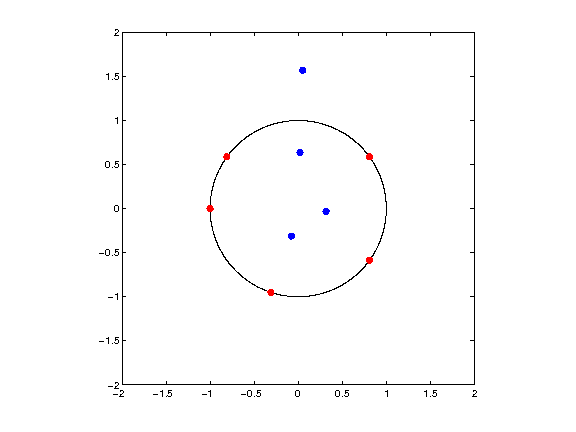Sort according to angle, and discard one out of two (because of double roots).

In :
[x1,I] = sort(mod(angle(R1),2*pi)/(2*pi));
x1 = x1(1:2:end);


Compare the found locations with the ground trust.

In :
disp( ['Error (should be 0): ' num2str(norm(x1-x0))] );

Error (should be 0): 3.1113e-09


## Noisy Recovery ¶

In practice, one has access to noisy observation $y=y_0+w$, and one approximates the support $x$ using the best $N$ local minima $\tilde x = \{\tilde x_1,\ldots,\tilde x_N\} \in \mathbb{T}^N$ of $d_{y}$.

Exercise 1

Compute and display $d_y$ VD _y isp

In :
exo1()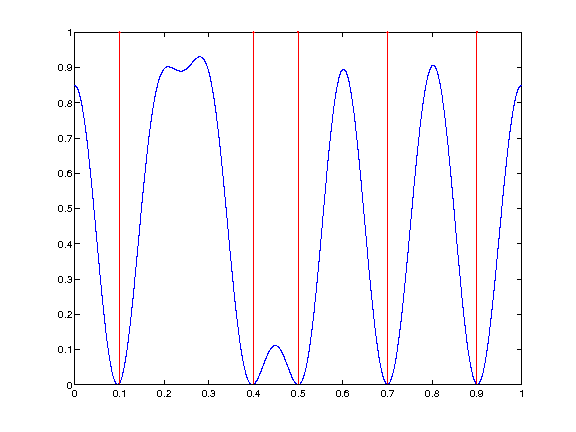In :
%% Insert your code here.


Exercise 2

Display the roots of $P_y$ that are inside the unit disk oefficients oots eep those inside isplay

In :
exo2()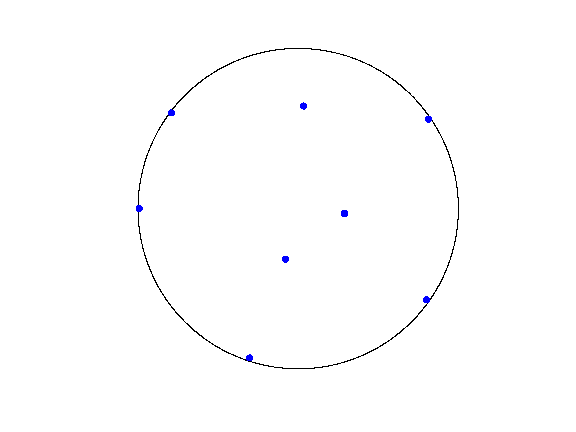In :
%% Insert your code here.


Exercise 3

Keep only the best $N$ ones, i.e. those that are the closest from the unit circle. We denote those as $\tilde x \in \mathbb{T}^N$. Recover an approximation $\tilde a$ of the amplitudes $a_0$ By solving in least squares (using backslash operator) the system $\Phi_{\tilde x} \tilde a = y.$ Display the recovered measure $m_{\tilde a, \tilde x}$. osition eep only the best N ones. ompute amplitude by solving a least square. isplay the recovered measure.

In :
exo3()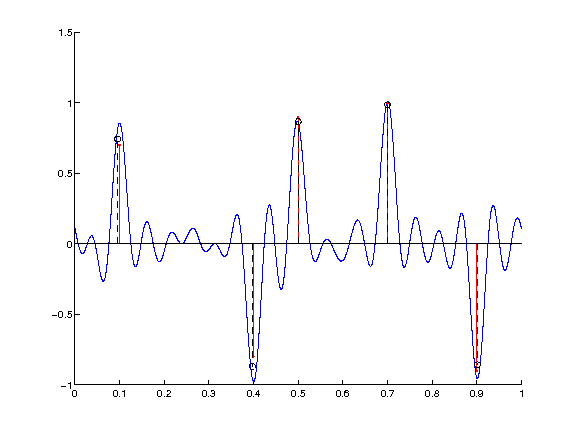In :
%% Insert your code here.


Exercise 4

Display the evolution of roots as the noise level $\sigma$ increases.

In :
exo4()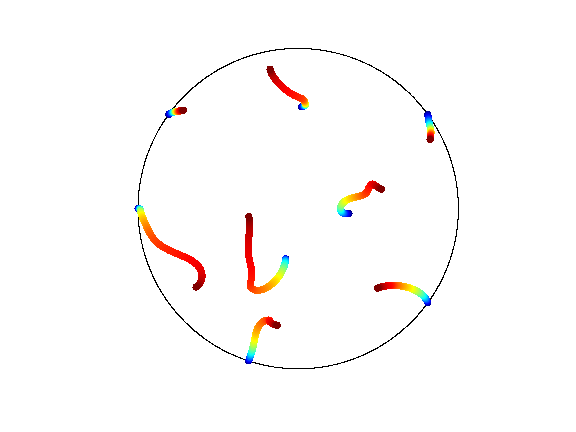In :
%% Insert your code here.


## Bibliography¶

• [Schmidt] R. Schmidt, Multiple emitter location and signal parameter estimation, IEEE Trans. on Antennas and Propagation, vol. 34, no. 3, pp. 276-280, 1986.
• [LiaoFannjiang14] W. Liao, A. Fannjiang, MUSIC for Single-Snapshot Spectral Estimation: Stability and Super-resolution, 2014.
• [Prony] G. R. B. de Prony, Essai Experimentale et Analytique, J. de L'Ecole Polytechnique 2, pp. 24-76, 1795.
• [KrimViberg] H. Krim and M. Viberg, Two decades of array signal processing research: the parametric approach, IEEE Signal Processing Magazine 13(4), pp.67-94, 1996.
• [RoyKailath] R. Roy and T. Kailath, ESPRIT ? Estimation of signal parameters via rotation invariance techniques, IEEE Trans. Acoust., Speech, Signal Proc., vol. 17, no. 7, July 1989
• [HuaSarkar] Y. Hua and T. K. Sarkar, Matrix pencil method for estimating parameters of exponentially damped/undamped sinusoids in noise, IEEE Transactions on Acoustics, Speech and Signal Processing 38(5), pp.814-824, 1990.
• [DemNeedNg] L. Demanet, D. Needell and N. Nguyen, Super-resolution via superset selection and pruning, Proceedings of the 10th International Conference on Sampling Theory and Applications, 2013.